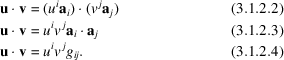International
Tables for
Crystallography
Volume B
Reciprocal space
Edited by U. Shumeli

International Tables for Crystallography (2010). Vol. B, ch. 3.1, p. 404   | 1 | 2 |

Section 3.1.2. Scalar product

D. E. Sandsa*

aDepartment of Chemistry, University of Kentucky, Chemistry–Physics Building, Lexington, Kentucky 40506–0055, USA
Correspondence e-mail: sands@pop.uky.edu

3.1.2. Scalar product

| top | pdf |

The scalar product of vectors u and v is defined aswhere u and v are the lengths of the vectors andis the angle between them. In terms of components,In all equations in this chapter, the convention is followed that summation is implied over an index that is repeated once as a subscript and once as a superscript in an expression; thus, the right-hand side of (3.1.2.4)implies the sum of nine termsThein (3.1.2.4)are the components of the metric tensor [see Chapter 1.1and Sands (1982a)]Subscripts are used for quantities that transform the same way as the basis vectors; such quantities are said to transform covariantly. Superscripts denote quantities that transform the same way as coordinates; these quantities are said to transform contravariantly (Sands, 1982a).

Equation (3.1.2.4)is in a form convenient for computer evaluation, with indices i and j taking successively all values from 1 to 3. The matrix form of (3.1.2.4)is useful both for symbolic manipulation and for computation,where the superscript italic T following a matrix symbol indicates a transpose. Written out in full, (3.1.2.6)isIf u is the column vector with components,is the corresponding row vector shown in (3.1.2.7).

References

Sands, D. E. (1982a). Vectors and Tensors in Crystallography. Reading: Addison Wesley. Reprinted (1995) Dover Publications.